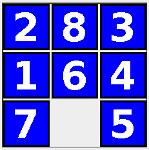This assignment has two main parts:
• You will add the 8-puzzle problem to the bridge crossing and water jug problems already supported by the problem solving framework
• You will modify the framework from the previous assignment so that it automatically solves problems using breadth-first or depth-first searching of the problem's state space
Click Program Behavior to the left to see how your program should look upon completion.

You will need to thoroughly understand:

Program requirements are divided between those for the 8-puzzle implementation and those for the automatic solving capability.
The implementation must extend the framework in a way analogous to the bridge crossing and water jug applications:
• PuzzleState implements State
• PuzzleMove extends Move
• PuzzleProblem extends Problem
• PuzzleCanvas extends Canvas
• Incorporate into the TestFrame class
However, you can eliminate (or simplify for testing) the PuzzleState.toString method.

For this assignment, the initial state will be the following state with a simple five-move solution:The RESET operation for the 8-puzzle problem should reset to this state.

The framework's GUI class must present the introduction, current state, move buttons, and reset button as before.

In addition, the requirements for an automatic problem solver are shown below.

Note that you may choose to lay out and present your GUI any way you like, provided these requirements are met:

• The user can elect to have the program solve the problem from any current state
• The user can choose between depth-first or breadth-first search of the problem state space
• Upon search completion, the following statistics are presented:
• Length of the solution from the current state, if found
• Number of DEQUE operations (adds and removes) performed during the search
• Maximum number of states stored on the DEQUE at any time during the search
• After a solution has been found, the user can:
• Click through the found solution one move at a time, or
• Observe all moves in the solution without interaction
Before you start, use NetBeans to copy your CS_2511_Framework_Graphics project from the previous assignment to a new NetBeans project called CS_2511_Solve (right click the project node in the Projects window and select Copy...).

In this section we give suggestions on how to implement the 8-puzzle and the automatic solver.

• Create a puzzle package under Source Packages in your CS_2511_Framework_Graphics NetBeans project for your 8-puzzle classes
• Decide on a state representation
• Don't need elaborate toString method
• You are encouraged, though it is not required, to write test classes for:
• PuzzleState: to test the equals method
• PuzzleMove: to test the doMove method
• PuzzleProblem: to test the success method
• Can implement a quick-and-dirty PuzzleCanvas by simply using Graphics2D.drawString to draw tile numbers on the component, then spend time beautifying the presentation later
• When your 8-puzzle implementation works, add it to the tabbed pane in TestFrame
The expand and search algorithms work on state space search tree vertices:

 ```Expand(u) children = {} for each move ∈ moves do child = move.doMove(u) if child ≠ null and not OccursOnPath(child, u) then d[child] = d[u] + 1 pred[child] = u add child to children return children ``` ```Search(s,adder) d[s] = 0 pred[s] = null DEQ = {s} while DEQ ≠ {} do u = Remove[DEQ] if success then return u else for each v ∈ Expand(u) do Add(DEQ,v,adder) return null ```

The graph package defines the types required by these algorithms: Vertex, SimpleVertex, and DequeAdder.

Install this package under Source Packages in your CS_2511_Framework_Graphics NetBeans project: graph.zip.

In order for State objects to act like graph vertices, state classes must extend the SimpleVertex class.

For example:

```package puzzle;

import framework.State;
import graph.SimpleVertex;

public class PuzzleState extends SimpleVertex implements State { ... }

```

Also for BridgeState and WaterJugState.

This part requires thorough understanding of Graph Search Implementation and State Space Search.
• Implement and test the expand algorithm — this was done in the lab exercise on state space search
• See the testExpand1 and testExpand2 methods in the ProblemTest class to the left
• Implement and test the search algorithm
• Put the search method in the Problem class along with expand
• Use a LinkedList which implements the Deque interface
• See the testSearch1 and testSearch2 methods in the ProblemTest class to the left
• Suggestion: when the final state is found, create an empty stack, push the final state, then follow predecessor links to the root, pushing states along the way. When the user wants to see the solution, this stack can be popped.
• Decide on a GUI layout for the added solving capability
• Create and add the needed GUI components
• Add component listeners where appropriate
In order to compute the required DEQ statistics:
• Initialize counters for queueOps, queueSize, and maxQueueSize before the search
• Increment queueOps each time DEQ is added to or removed from
• Decrement queueSize each time DEQ is removed from
• Increment queueSize each time DEQ is added to, and set maxQueueSize to max(queueSize, maxQueueSize)
• Display queueOps and maxQueueSize in the GUI upon search completion.
To test your expand and search algorithms, install the JUnit test class shown below.

• Right-click your framework's Problem class, choose Tools, and then Create JUnit Tests
• Replace the generated code with that shown below